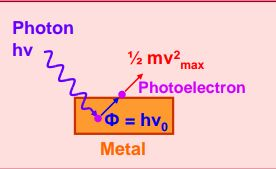Q

# Can someone explain When the light of frequency 2v0 (where v0 is threshold frequency), is incident on a metal plate, the maximum velocity of electrons emitted is v1. When the frequency of the incident radiation is increased to 5v0, the maximum veloci

When the light of frequency 2v0 (where v0 is threshold frequency), is incident on a metal plate, the maximum velocity of electrons emitted is v1. When the frequency of the incident radiation is increased to 5v0, the maximum velocity of electrons emitted from the same plate is v2 . The ratio of v1 to v2 is

• Option 1)

4:1

• Option 2)

1:4

• Option 3)

1:2

• Option 4)

2:1

256 Views

As we have learned

Conservation of energy -

- whereinFrom einstein equation

Initially

finally

or

Option 1)

4:1

This is incorrect

Option 2)

1:4

This is incorrect

Option 3)

1:2

This is correct

Option 4)

2:1

This is incorrect

Exams
Articles
Questions